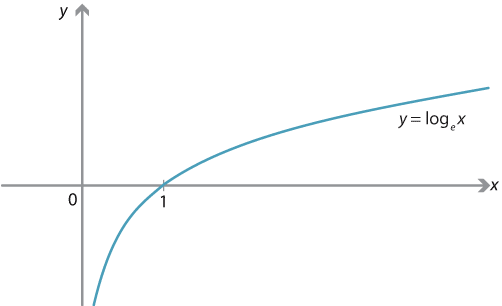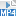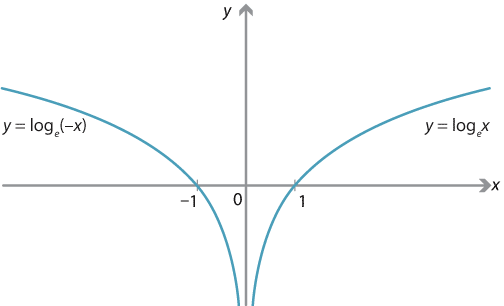## Content

### The natural logarithm

The logarithm to the base $$e$$ is an important function. It is also known as the natural logarithm. It is defined for all $$x>0$$:

$y = \log_e x \ \iff \ x = e^y.$

The alternative notation $$\ln x$$ (pronounced `ell-en' $$x$$) is often used instead of $$\log_e x$$.Graph of $$y = \log_e x$$ or, equivalently, $$x = e^y$$.
Detailed description

Since we know how to differentiate the exponential, we can now also differentiate the natural logarithm. If $$y = \log_e x$$, then $$x=e^y$$, so

$\dfrac{dx}{dy} = e^y = x.$

Now, by the chain rule, $$1 = \dfrac{dy}{dy} = \dfrac{dy}{dx} \dfrac{dx}{dy}$$, and so

$\dfrac{dy}{dx} = \dfrac{1}{\dfrac{dx}{dy}} = \dfrac{1}{x}.$

Therefore, we conclude that the derivative of $$f(x) = \log_e x$$ is $$f'(x) = \dfrac{1}{x}$$.

Exercise 3

Give an alternative proof that the derivative of $$\log_e x$$ is $$\dfrac{1}{x}$$, by differentiating both sides of the equation

$x = e^{\log_e x}.$

Screencast of exercise 3Knowing the derivative of $$\log_e x$$ allows us to differentiate many related functions.

#### Example

Find the derivative of $$f(x) = \log_e (2x+5)$$.

#### Solution

The chain rule gives

$f'(x) = \dfrac{1}{2x+5} \cdot 2 = \dfrac{2}{2x+5}.$

In general, for any real constants $$a$$ and $$b$$ with $$a \neq 0$$, we can consider the function $${f(x) = \log_e(ax+b)}$$. Its derivative is, again using the chain rule,

$f'(x) = \dfrac{a}{ax+b}.$

A warning about domains. Any logarithm function $$\log_a x$$, with base $$a>1$$, is defined only for $$x>0$$; its domain is $$(0, \infty)$$. Yet the function $$\dfrac{1}{x}$$ is defined for all $$x \neq 0$$, including all negative $$x$$. Strictly speaking, the derivative of $$\log_e x$$ is the function $$\dfrac{1}{x}$$, restricted to the domain $$(0, \infty)$$.

In the previous example, $$\log_e(2x+5)$$ is only defined when $$2x+5>0$$, that is, $$x > - \dfrac{5}{2}$$; so the functions $$f$$ and $$f'$$ both have domain $$(-\dfrac{5}{2}, \infty)$$.

Exercise 4

Consider the function $$f \colon (-\infty, 0) \to \mathbb{R}$$ defined by $$f(x) = \log_e (-x)$$. Show that $$f'(x) = \dfrac{1}{x}$$, restricted to the domain $$(-\infty, 0)$$.

Using the preceding exercise, we can construct a function which is defined for all $$x \ne 0$$, and whose derivative is always $$\dfrac{1}{x}$$. We define $$f \colon \mathbb{R} \backslash \{0\} \to \mathbb{R}$$ as

$f(x) = \log_e |x| = \begin{cases} \log_e x &\text{if $$x > 0$$}\\ \log_e (-x) &\text{if $$x < 0$$.} \end{cases}$

As both $$\log_e x$$ and $$\log_e(-x)$$ have derivative $$\dfrac{1}{x}$$, we conclude that $$f'(x) = \dfrac{1}{x}$$ for all $$x \neq 0$$.Graph of $$y = \log_e |x|$$, which is the union of the graphs
$$y = \log_e x$$, for $$x > 0$$, and $$y = \log_e(-x)$$, for $$x < 0$$.
Detailed description

Exercise 5

What is the domain of the function $$f(x) = \log_2 (3-7x)$$?

### The derivative of $$2^x$$, revisited

We now obtain a simple answer to our original question.

#### Example

What is the derivative of $$f(x) = 2^x$$?

#### Solution

Since $$2 = e^{\log_e 2}$$, we have $$f(x) = e^{\log_e 2 \cdot x}$$. Using the chain rule, we obtain

\begin{align*} f'(x) &= \log_e 2 \cdot e^{\log_e 2 \cdot x}\\ &= \log_e 2 \cdot 2^x. \end{align*}

Previously we found that $$f'(x) \approx 0.693147 \cdot 2^x$$. We now see that the constant is $$\log_e 2$$.

Exercise 6

Find the derivatives of the following functions:

1. $$f(x) = 2^{x^2}$$
2. $$f(x) = 3^{4x^2 + 2x - 7}$$.

Exercise 7

Use what we've done so far to explain why

$\lim_{h \to 0} \dfrac{2^h - 1}{h} = \log_e 2.$

Next page - Content - Derivatives of general exponential functions